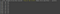# Day 9: Finding “fun” parallels between MATLAB and Python

I used MATLAB regularly for five years, but I didn’t really feel proficient until I started using it the way I use Python.

There are two things that I find myself doing in Python constantly:

1. Using list comprehensions: This allows you to loop through a variable that has multiple entries (ie. [1,2,3,4]), and have a function operate on each element. In Python, this is done through a nice and compact “list comprehension”. In MATLAB, the fun family serve this purpose.

In my previous days of ‘30 days of MATLAB tips I wish I had known doing graduate school in neuroscience’, I covered various uses of the fun family to operate down through single arrays, cell arrays, or rows in tables. In this post, I’ll focus on looping through “zipped” variables by using the fun family.

## Let’s start by manipulating text by numbers

Use the code below to generate the table shown. You may have this type of table for your experiments, for example, if there are different groups of test subjects and different conditions (I only show the first four rows for brevity):

`rng(5)mytable = table( randi(10,26,1), char(65:90)', 'VariableNames', {'Number','Letter'} );`

The table will basically contain a number in one column and a letter in the other. Note that these are very different variable types! Combining these data types in a useful way is a notoriously complex task in MATLAB (and many coding languages).

Tip: To generate letters A-Z in a column, use char(65:90)’. Use char(97:122)’ if you want uppercase.

To show off how rowfun can accomplish such a “zipping” of two very different variable types.

`output = rowfun( @(Number,Letter) repmat(Letter,1,Number), mytable, 'OutputFormat', 'cell' );`

Just to break it down, what we’ve done here is say apply the anonymous function:

`@(Number,Letter) repmat(Letter,1,Number)`

To the variables Number and Letter, where the variables are coming from mytable. In effect, “tabling” could be considered analogous to zipping!

This was a convenient way to manipulate the string without having to separately index into the two columns of mytable.

Let’s extend it to three columns.

`rng(5)mydata = rand(10,100); % Lets say you have ten samples, 100 time points for each samplemytable = table( [1*ones(5,1);2*ones(5,1)], [char(65:69)';char(65:69)'], mydata, 'VariableNames', {'Group','Letter','Data'} );`

Here’s your table containing three columns, each column is basically an array containing useful values that you’ll want to combine.

Using this table, you can combine all three columns to produce a summary of your data:

`rowfun( @(Number,Letter,Data) fprintf( 'Group %i (%s) %1.2f\n',Number,Letter,mean(Data) ) , mytable);`Making a little summary of your data is easy if you have it in a table.

There are several caveats to consider when using tables with rowfun, which I will get into in future posts. Stay tuned.

Neuroscientist and data scientist at Columbia University. On Twitter: @NeuroJoJo

## More from Neurojojo

Neuroscientist and data scientist at Columbia University. On Twitter: @NeuroJoJo